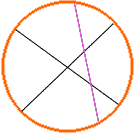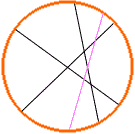What is the greatest number of pieces of pizza you can form if you use five straight cuts to cut the pizza? answer given is 16. please draw a diagram of the answer. thanks, woody Hi Woody, I am going to try to develop an expression that tells you "the greatest number of pieces of pizza you can form if you use k straight cuts to cut the pizza". I want to call it p(k), so p(1) =2 (2 pieces with 1 cut), and p(2) = 4. (I suppose also the p(0) = 1, zero cuts leaves the pizza in 1 piece.) Now to make the third cut you want to make sure that it intersects each previous cut because each time you intersect a cut you produce a new piece. Also you clearly don't want to cut through the point where the previous cuts intersect. Hence, from the diagram, p(3) = 7. From the diagram you can see how the 7 arises, p(3) = p(2) + 3, that is the third cut intersected the previous 2 cuts and produced 3 additional pieces.You want the the fourth cut to intersect all three previous cuts and hence produce 4 additional pieces so p(4) = p(3) + 4 = 7 + 4 = 11. In general the recursive expression is p(k) = p(k-1) + k.Cheers, Penny Go to Math Central To return to the previous page use your browser's back button.